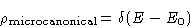Subsections

# Time Evolution and Ergodicity

## Gibbs Postulate

Trajectory--a line in phase space. Many-body systems: a complex entangled trajectory. Assumption: after long time the system forgets'' the initial conditions. Probability of each state does not depend on where we started!

What can it depend upon? Energy, total momentum, total volume,...

Microcanonical Ensemble:
Closed system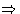all states have same energy, momentum,...
Gibbs Postulate:
All states in microcanonical ensemble are equivalent. They have the same probability to occur.

## Averaging and Ergodicity

Two ways of averaging:
1.
I take one system and observe it for a long time (time averaging) :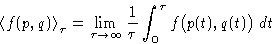(1)
2.
I take many systems from my ensemble (i.e. with same energy) and observe them all in one time (ensemble average):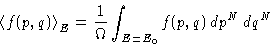(2)
with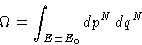(3)

Ergodicity hypothesis: ensemble average is equal to time average.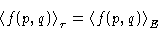Is ergodic hypothesis alway correct?

Gibbs postulate: yes.

But how large should be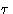in (1) for the limit to work? What happens if it is a year? Twenty years? The mountains flowed down at Thy presence, but we are mortals....

Examples: water is liquid at frequencies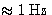, but solid at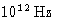. Window panes flow in hundred years...

Ergodic systems:is small, and ergodicity works. Gases, liquids, crystals.
Non-ergodic systems:is large, and we cannot wait for equilibrium. Glasses.
The same system can be both ergodic and non-ergodic depending on your time scale. Polymers.

Computer simulations: is the simulated system ergodic? Do we study its equilibrium or transient features?

## Entropy

Each system in the ensemble is a point in the phase space. How many points are there?

• Discrete space--just count them!
• Continuous space: measure the volume! Number of states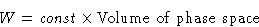Thecannot be obtained in classical statistics. Quantum answer: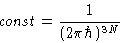Geometric interpretation: cell model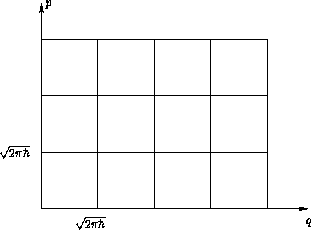Entropy: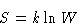(4)
Boltzmann constant: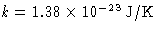## Thermodynamic limit

Suppose we increase the volume of our system V and the number of particles N.

Thermodynamic limit (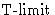):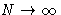,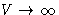,and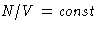. This limit is always taken!
Intensive variables:
A variable A is intensive if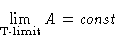Examples: temperature, pressure, density, concentration,...
Extensive variables:
A variable A is intensive, if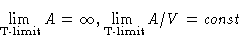Examples: number of particles, volume, energy,...

Entropy of two non-interacting subsystems: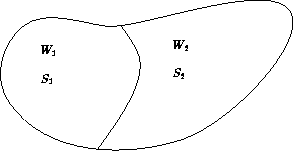The total number of states: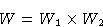Entropy:

S = S1 + S2

Thermodynamic limit: many weakly interacting parts!
Entropy is an extensive quantity

## Distribution Function

Let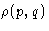be the probability to meet the given state in the ensemble. Ensemble averaging: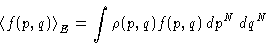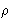is the distribution function . It depends on the ensemble. Normalization: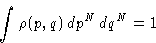(5)
Question:
What is the distribution function for the microcanonical ensemble?
It is zero if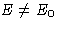, and satisfies (5). Therefore